# 详解十大经典机器学习算法——EM算法

2019/04/10 10:10

<br>

EM算法的英文全称是Expectation-maximization algorithm，即最大期望算法，或者是期望最大化算法。EM算法号称是十大机器学习算法之一，听这个名头就知道它非同凡响。我看过许多博客和资料，但是少有资料能够将这个算法的来龙去脉以及推导的细节全部都讲清楚，所以我今天博览各家所长，试着尽可能地将它讲得清楚明白。

## 最大似然估计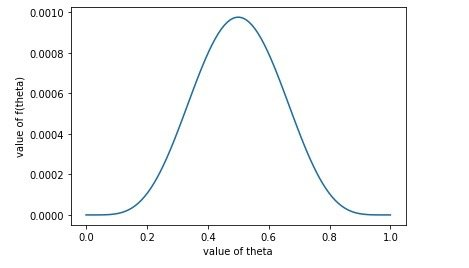## 引入隐变量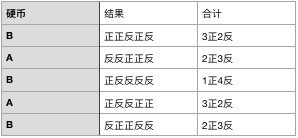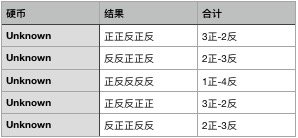EM算法正是为了解决这个问题诞生的。

## EM算法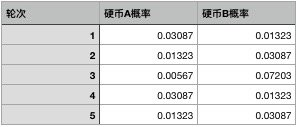## 例子改进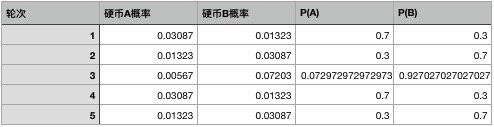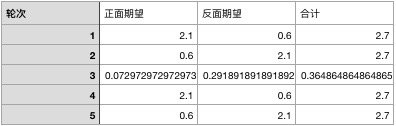$p_1 = \frac{2.1+0.6+0.0729+2.1+0.6}{2.7+2.7+0.292+2.7+2.7}=0.490$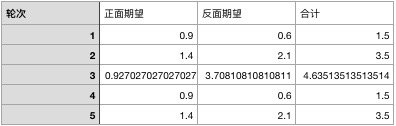## 数学证明

$P_i = P(x_i, z_i; \theta)$

$\sum_{i=1}^m\log P_i= \sum_{i=1}^m \log \sum_{z_i}P(x_i, z_i; \theta)$

$\sum_{i=1}^m\log P_i= \sum_{i=1}^m \log \sum_{z_i}Q_i(z_i)\frac{P(x_i, z_i; \theta)}{Q_i(z_i)}$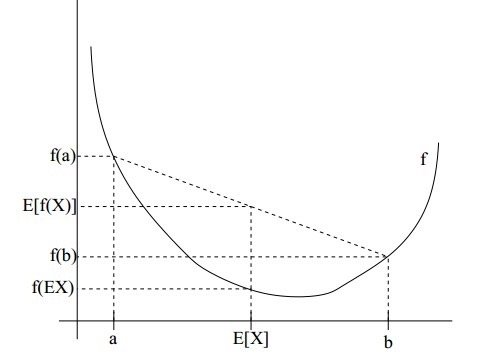$\sum_{i=1}^m\log P_i \geq \sum_{i=1}^m \sum_{z_i}Q_i(z_i)\log \frac{P(x_i, z_i; \theta)}{Q_i(z_i)}$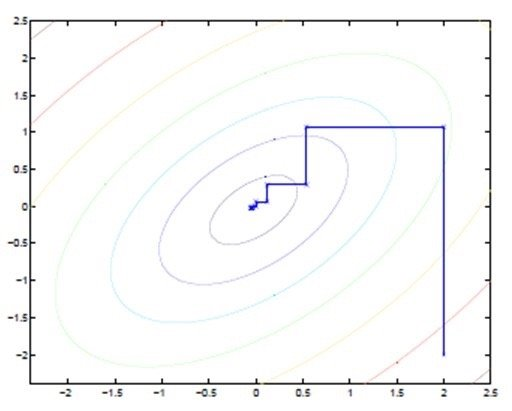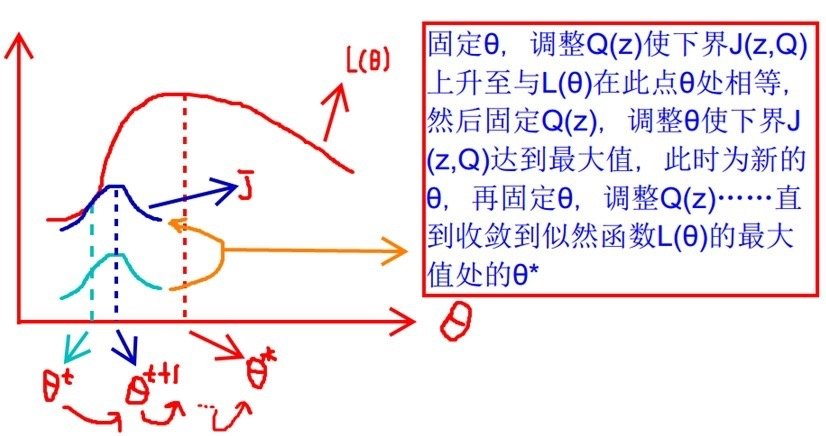$\frac{P(x_i, z_i, \theta)}{Q_i(z_i)} = c$

\begin{aligned} Q_i(z_i)\cdot c &= P(x_i, z_i, \theta) \ Q_i(z_i) &=\frac{P(x_i. z_i; \theta)}{c}\ Q_i(z_i) &= \frac{P(x_i. z_i; \theta)}{\sum_{z_i}P(x_i, z_i; \theta)}\ Q_i(z_i) &= \frac{P(x_i. z_i; \theta)}{P(x_i; \theta)}\ Q_i(z_i) &= P(z_i|x_i; \theta)\ \end{aligned}

$L(\theta_t) = \sum_{i=1}^m \sum_{z_i}Q_i(z_i)\log \frac{P(x_i, z_i; \theta_t)}{Q_i(z_i)}$

\begin{aligned} L(\theta_{t+1}) &\geq \sum_{i=1}^m \sum_{z_i}Q_i(z_i)\log \frac{P(x_i, z_i; \theta_{t+1})}{Q_i(z_i)}\ &\geq \sum_{i=1}^m \sum_{z_i}Q_i(z_i)\log \frac{P(x_i, z_i; \theta_{t})}{Q_i(z_i)} \ &=L(\theta) \end{aligned}

## 总结0
0 收藏

### 作者的其它热门文章0 评论
0 收藏
0